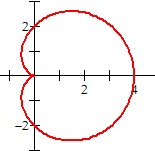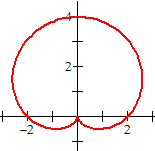# cardioid

Cardioid

A curve that is somewhat heart shaped. A cardioid can be drawn by tracing the path of a point on a circle as the circle rolls around a fixed circle of the same radius. The equation is usually written in polar coordinates.

Note: A cardioid is a special case of the limaçon family of curves.

Cardioid:   r = a ± a cos θ (horizontal) or  r = a ± a sin θ (vertical)r = 2 + 2cos θr = 2 + 2sin θ